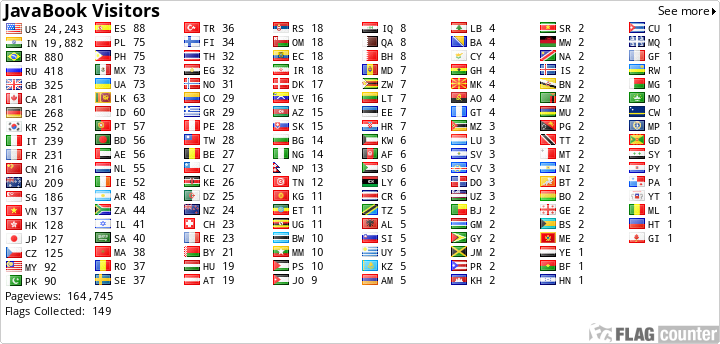## ContactUs

### JDBC : What are Query methods ?

1.If we are executing the aggregate functions then always the actual result will be 1 row.
"select count(SNO) from STUDENT".
If we execute the above query, the expected type is "int" and the expected rows are 1 and the columns are 1. In this case to execute the above query we can use the "queryForInt()", which returns 1 row and 1 column with the int type.
queryForInt() ==> 1 Row ==> 1 Column ==> int

2. String query = "select avg(SNO) from STUDENT";
The above query expected type is "Float" and to execute the above query we can use the "queryForObject()" and we need to pass the type.
queryForObject() ==> 1 Row ==> 1 Column ==> any type

3.String query = "select count(SNO),avg(SNO) from STUDENT";
If we execute the above query then the expected rows are 1 and the expected columns are 2.
We can use the "queryForMap()" to execute the above query.
queryForMap() ==> 1 Row ==> N Columns ==>any type

Note : If the actual result is 0 or more than 1 row then it will throw the exception[InCorrectResultSizeDataAccessException].

If the actual result is 0 or more than 1 row, most of the cases we never use queryForInt()/queryForObject()/queryForMap().....we use RowMapper concept.